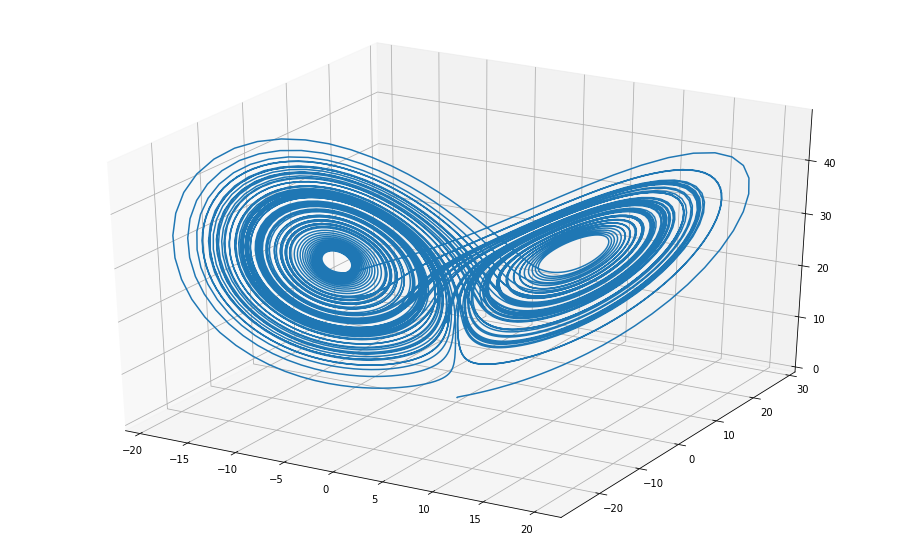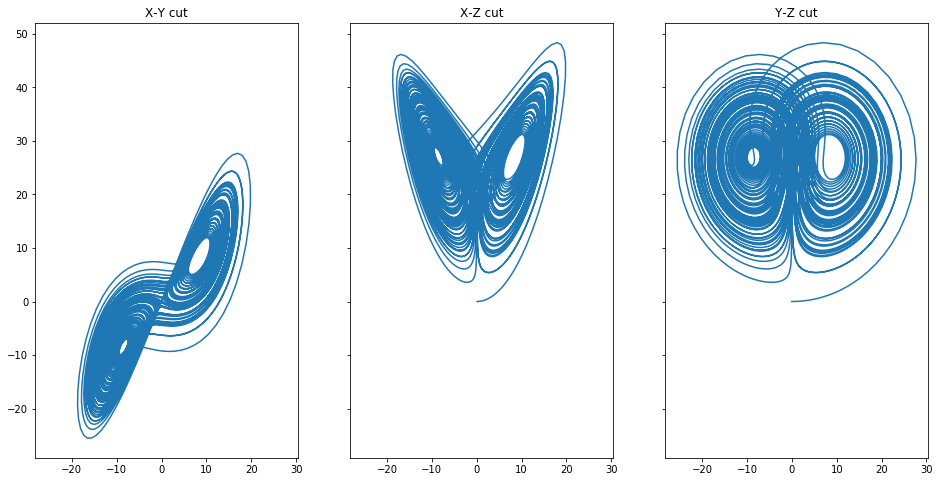# Lorenz Attractor¶

by Paulo Marques, 2013/09/21

This notebook implements the beautiful Lorenz Attractor in Python. The Lorenz Attractor is probably the most ilustrative example of a system that exibits cahotic behaviour. Slightly changing the initial conditions of the system leads to completely different solutions. The system itself corresponds to the movement of a point particle in a 3D space over time.

The system is formally described by three different differential equations. These equations represent the movement of a point $(x, y, z)$ in space over time. In the following equations, $t$ represents time, $\sigma$, $\rho$, $\beta$ are constants.

$$\frac{dx}{dt} = \sigma (y - x)$$$$\frac{dy}{dt} = x (\rho - z) - y$$$$\frac{dz}{dt} = x y - \beta z$$

Let's implement it in python.

Let's start by importing some basic libraries.

In :
%matplotlib inline
from scipy.integrate import odeint
from mpl_toolkits.mplot3d.axes3d import Axes3D
from pylab import *


We need to define the system of differential equations as an equation of the form: ${\bf r}' = {\bf f}({\bf r},t)$ where ${\bf r} = (x, y, z)$ and ${\bf f}({\bf r},t)$ is the mapping function.

In :
def f(r, t):
(x, y, z) = r

# The Lorenz equations
dx_dt = sigma*(y - x)
dy_dt = x*(rho - z) - y
dz_dt = x*y - beta*z

return [dx_dt, dy_dt, dz_dt]


Let's define the initial conditions of the system ${\bf r}_0 = (x_0, y_0, z_0)$, the constants $\sigma$, $\rho$ and $\beta$ and a time grid.

In :
# Initial position in space
r0 = [0.1, 0.0, 0.0]

# Constants sigma, rho and beta
sigma = 10.0
rho   = 28.0
beta  = 8.0/3.0

# Time grid
tf = 100.0
t = linspace(0, tf, int(tf*100))


Now let's solve the differencial equations numericaly and extract the corresponding $(x, y, z)$:

In :
pos = odeint(f, r0, t)

x = pos[:, 0]
y = pos[:, 1]
z = pos[:, 2]


Let's see how it looks in 3D.

In :
fig = figure(figsize=(16,10))
ax = fig.gca(projection='3d')
ax.plot(x, y, z)

Out:
[<mpl_toolkits.mplot3d.art3d.Line3D at 0x116f80f60>]Let's see different cuts around the axes:

In :
fig, ax = subplots(1, 3, sharex=True, sharey=True, figsize=(16,8))

ax.plot(x, y)
ax.set_title('X-Y cut')

ax.plot(x, z)
ax.set_title('X-Z cut')

ax.plot(y, z)
ax.set_title('Y-Z cut')

Out:
Text(0.5, 1.0, 'Y-Z cut')